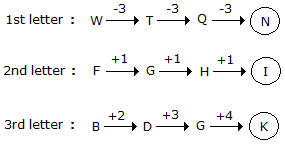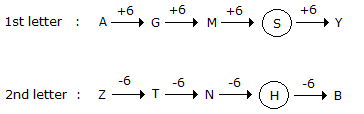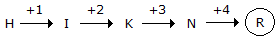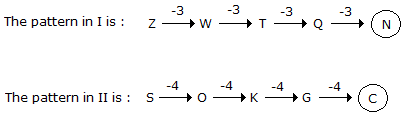# Verbal Reasoning - Series Completion

### Exercise :: Series Completion - Section 4

In each of the following questions, various terms of an alphabet series are given with one or more terms missing as shown by (?). Choose the missing terms out of the given alternatives.

1.

WFB, TGD, QHG, ?

 A. NIJ B. NIK C. NJK D. OIK E. PJK

Explanation:2.

AZ, GT, MN, ?, YB

 A. JH B. SH C. SK D. TS

Explanation:3.

H, I, K, N, ?

 A. O B. Q C. R D. S

Explanation:4.

Z, S, W, O, T, K, Q, G, ?, ?

 A. N, C B. N, D C. O, C D. O, D

Explanation:

The given sequence is a combination of two series:

I. Z, W, T, Q, ? and II. S, O, k, G, ?5.

b e d f ? h j ? l

 A. i m B. m i C. i n D. j m

Explanation:

The series may be divided into groups as shown:

b e d / f ? h / j ? l

Clearly in the first group, the second and third letters are respectively three and two steps ahead of the first letter.

A similar pattern would follow in the second and third groups.